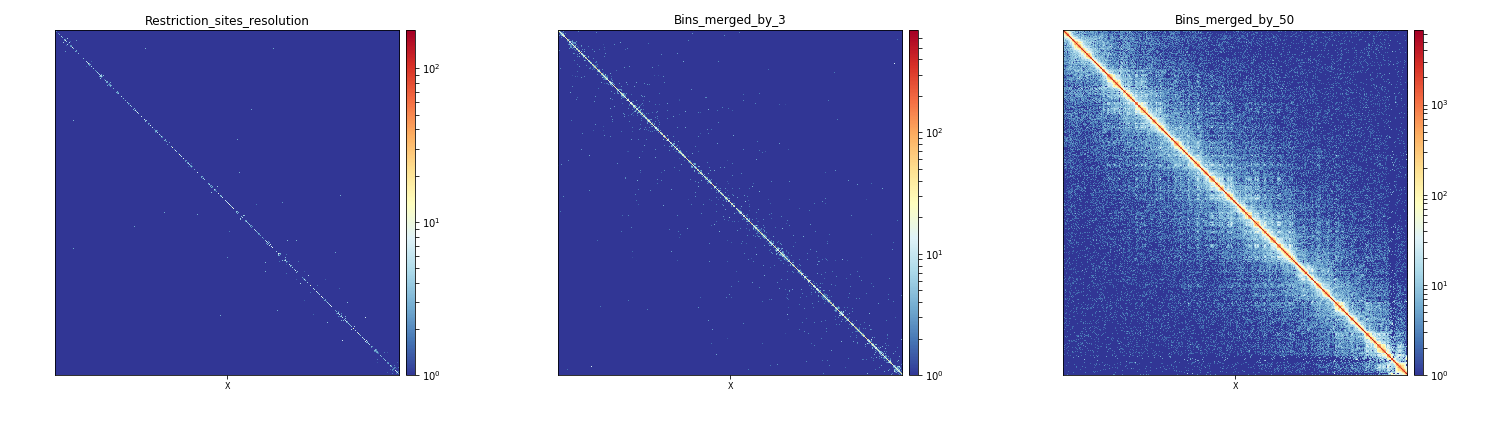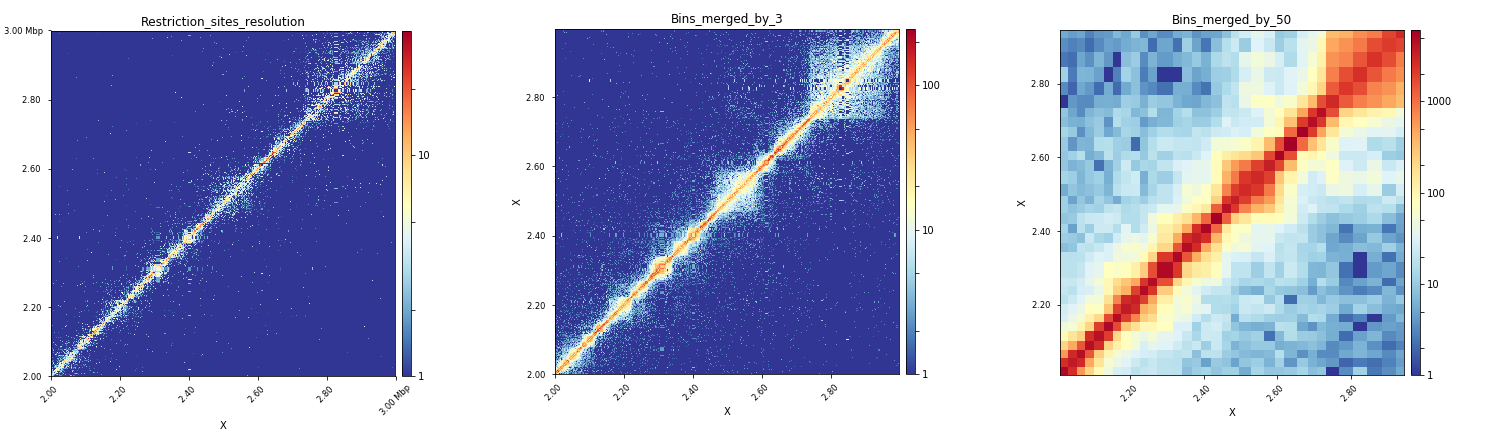# hicMergeMatrixBins¶

## Background¶

Depending on the downstream analyses to perform on a Hi-C matrix generated with HiCExplorer, one might need different bin resolutions. For example using hicPlotMatrix to display chromatin interactions of a whole chromosome will not produce any meaningful visualization if it is performed on a matrix at restriction sites resolution. hicMergeMatrixBins address this issue by merging a given number of adjacent bins (determined by `--numBins`). To minimize the loss of information (matrix bins with few counts), it is recommended to perform hicMergeMatrixBins on uncorrected matrices (before matrix correction). The matrix correction can be performed after bin merging for downstream analyses using hicCorrectMatrix.

## Description¶

Merges bins from a Hi-C matrix. For example, using a matrix containing 5kb bins, a matrix of 50kb bins can be derived using –numBins 10. From one type of downstream analysis to another, different bin sizes are used. For example to call TADs, unmerged matrices are recommended while to display Hi-C matrices, bins of approximately 2000bp usually yield the best representations with hicPlotMatrix for small regions, and even larger bins (50kb) are recommended for whole chromosome representations or for hicPlotDistVsCounts.

```usage: hicMergeMatrixBins --matrix matrix.h5 --outFileName OUTFILENAME
--numBins int [--runningWindow] [--help] [--version]
```

### Required arguments¶

--matrix, -m

Matrix to reduce in h5 format.

--outFileName, -o

File name to save the resulting matrix. The output is also a .h5 file. But don’t add the suffix.

--numBins, -nb

Number of bins to merge.

### Optional arguments¶

--runningWindow

Set to merge for using a running window of length –numBins.

Default: False

--version

show program’s version number and exit

## Usage example¶

### Running hicMergeMatrixBins¶

Below, we display the example of plotting a Hi-C matrix at the scale of the whole X-chromosome and at the scale of a 1Mb region of the X chromosome. To do this, we will perform two different bin merging using hicMergeMatrixBins on an uncorrected matrix built at restriction site resolution using hicBuildMatrix. To do this, we run the two following commands:

```\$ hicMergeMatrixBins -m myMatrix.h5 -o myMatrix_merged_nb3.h5 -nb 3

\$ hicMergeMatrixBins -m myMatrix.h5 -o myMatrix_merged_nb50.h5 -nb 50
```

Starting from a matrix `myMatrix.h5` with bins of a median length of 529bp, the first command produces a matrix `myMatrix_merged_nb3.h5` with bins of a median length of 1661bp while the second command produces a matrix `myMatrix_merged_nb50.h5` with bins of a median length of 29798bp. This can be checked with hicInfo.

After correction of these three matrices using hicCorrectMatrix, we can now plot the corrected matrices `myMatrix_corrected.h5`, `myMatrix_merged_nb3_corrected.h5` and `myMatrix_merged_nb50_corrected.h5` at the scale of the whole X-chromosome and at the X:2000000-3000000 region to see the effect of bin merging on the interactions visualization.

### Effect of bin merging at the scale of a chromosome¶

```\$ hicPlotMatrix -m myMatrix_corrected.h5 \
-o myMatrix_corrected_Xchr.png \
--chromosomeOrder X \
-t Restriction_sites_resolution --log1p \

\$ hicPlotMatrix -m myMatrix_merged_nb3_corrected.h5 \
-o myMatrix_merged_nb3_corrected_Xchr.png \
--chromosomeOrder X \
-t Bins_merged_by_3 --log1p \

\$ hicPlotMatrix -m myMatrix_merged_nb50_corrected.h5 \
-o myMatrix_merged_nb50_corrected_Xchr.png \
--chromosomeOrder X \
-t Bins_merged_by_50 --log1p \
```

When observed altogether, the plots produced by these three commands show that merging of bins by 50 is the most adequate way to plot interactions for a whole chromosome in Drosophila melanogaster when starting from a matrix with bins of a median length of 529bp.### Effect of bin merging at the scale of a specific region¶

``` \$ hicPlotMatrix -m myMatrix_corrected.h5 \
-o myMatrix_corrected_Xregion.png \
--region X:2000000-3000000 \
-t Restriction_sites_resolution --log1p \

\$ hicPlotMatrix -m myMatrix_merged_nb3_corrected.h5 \
-o myMatrix_merged_nb3_corrected_Xregion.png \
--region X:2000000-3000000 \
-t Bins_merged_by_3 --log1p \